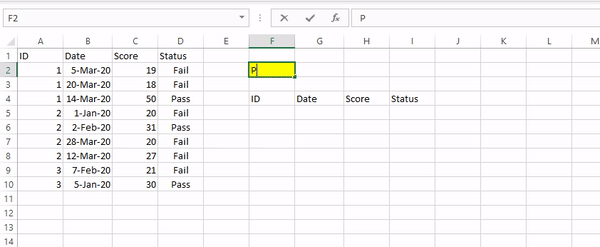top of page
Search

# VLOOKUP with conditionsI have been working & training on MS Excel for quite some time, and VLOOKUP has been one of the most popular formulas. Most of us who deal with data should figure out ways to add more details in an existing table, especially taking reference from another table. VLOOKUP has a proven track record in this context.

By default, VLOOKUP returns the search result based on one matching column. But at times, we need to match more than one condition and then return the expected result.

How can we achieve this without adding any helper column? In modern Excel, there are multiple ways of accomplishing this. In this article, we use the FILTER function.

In the past few months, Office 365 has seen the inclusion of array-based formulas. FILTER is one of them.

## Decoding FILTER function

FILTER has the following syntax:

FILTER(array,include,[if_empty])

· Array: The range which we need in return

· Include: The column which we would like to filter

· [if_empty] (Optional): Values to return if no matches found

Let’s consider the following example:We can use the following formula in cell F5

`=FILTER(A1:D10,D1:D10=F3,"")`And it returns a table with the matching Status.FILTER function returns a range meeting conditions

## Using it with VLOOKUP

Let’s say we need to find only those records where the Status = “Pass”. The VLOOKUP formula is:

`=VLOOKUP(\$F3,FILTER(\$A\$1:\$D\$10,\$D\$1:\$D\$10="Pass",""),2,TRUE)`Using FILTER function, we have filtered the array to return only results with Status = “Pass”, and then VLOOKUP formula does the rest.We can combine more multiple conditions with * (which works as AND operator)